Related Articles

# Class 9 NCERT Solutions – Chapter 1 Number System – Exercise 1.4

• Last Updated : 09 Mar, 2021

### Question 1. Visualize 3.765 on the number line, using successive magnification.

Solution:

Now in the given question, you have to represent 3.765 on the number line using the successive magnification method.

Step 1: We will check the range of the number and magnify it accordingly. That is, 3.765 will lie between 3 and 4 so we will magnify the value between 3 and 4.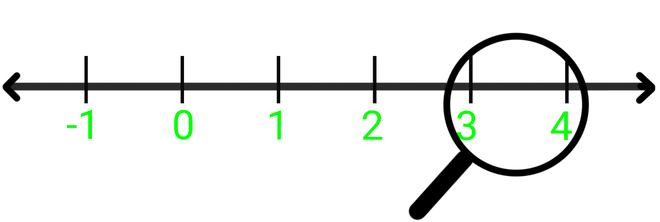Magnify 3 and 4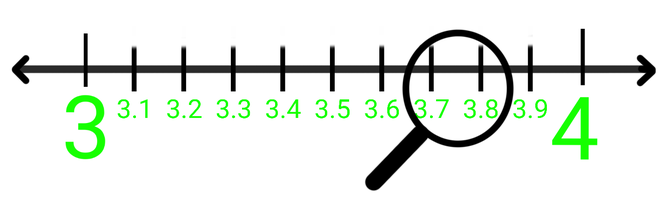Step 2: Now 3.76 will lie between 3.7 and 3.8, so we will magnify the value between 3.7 and 3.8.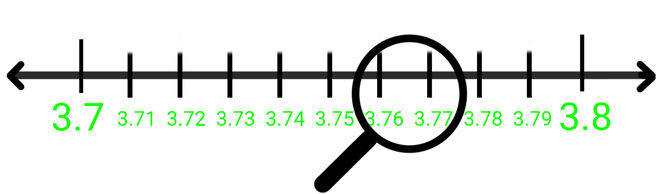Step 3: Now as we will magnify we will check the range, that is, 3.765 will lie between 3.76 and 3.77.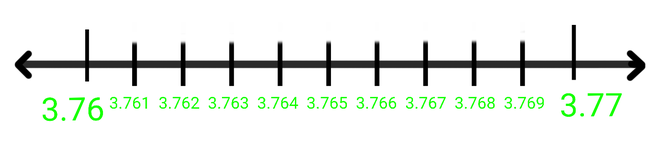Step 4: Now as we will magnify the value between 3.76 and 3.77 we will find the value 3.765.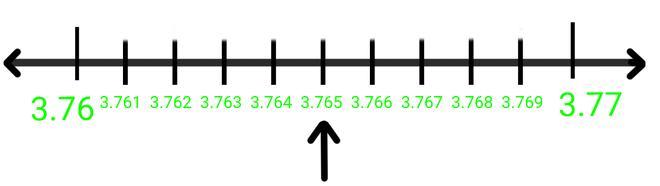Hence 3.765 is found between 3.76 and 3.77

### Question 2. Visualizeon the number line, up to 4 decimal places.

Now in this question, we have to represent the numberon the number line. The bar sign (   ͞   ) above .26 depicts that the number .26 is repeated continuously after the decimal point.  But it is given that we have to represent the number up to 4 decimal places only, that is, we have to represent 4.2626 on the number line.

Step 1: We will check the range of the number and magnify it accordingly.

Step 2: Now 4.2626 will lie between 4 and 5, so we will magnify the value between 4 and 5.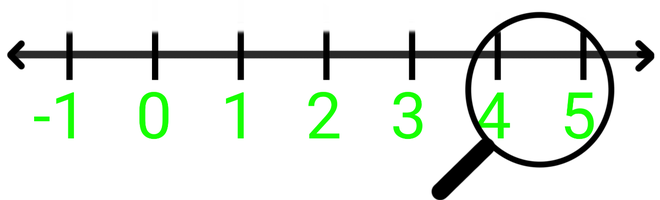Magnify 4 and 5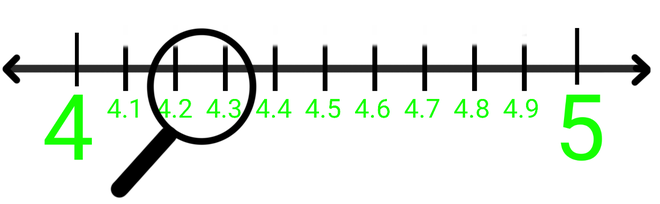Step 3: 4.26 will lie between 4.2 and 4.3, so we will magnify the value between 4.2 and 4.3.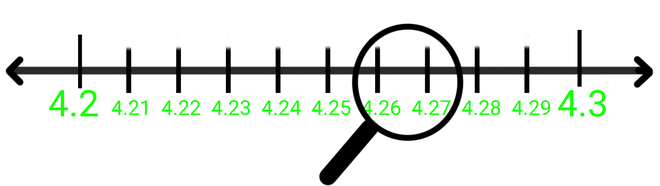Step 4: 4.262 will lie between 4.26 and 4.27, so we will magnify the value between 4.26 and 4.27.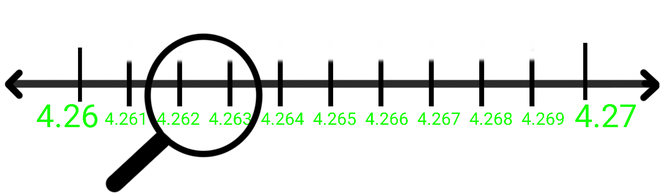Step 5: Now at last 4.2626 will lie between 4.262 and 4.263, so we will magnify the value between 4.262 and 4.263.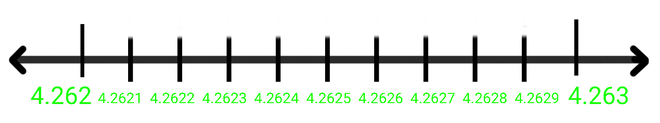Step 6: As we will magnify the value between 4.262 and 4.263 we will get 4.2626.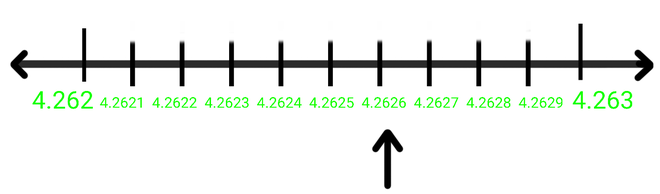Hence 4.2626 is found between 4.262 and 4.263

Attention reader! Don’t stop learning now. Participate in the Scholorship Test for First-Step-to-DSA Course for Class 9 to 12 students.

My Personal Notes arrow_drop_up